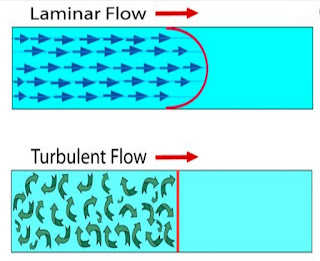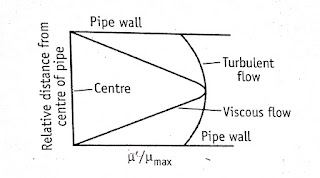Type Here to Get Search Results !
×
Hi ,

We always try to serve you better. Please enter the institute name, you belong to.

×
Hello ,

# Flow of Fluids - Reynolds Number, Pharmaceutical Engineering, B.Pharmacy As per PCI Syllabi

## Flow of Fluids

### Content: Types of manometers, Reynolds numbers and its significance, Bernoulli's theorem and its applications. Energy losses, Orifice meter, Venturimeter, Pitot tube and Rotameter.

Part 1:- Types of manometers
Part 2:- Reynolds numbers and its significance
Part 3:- Bernoulli's theorem and its applications
Part 4:- Energy losses, Orifice meter, Venturimeter, Pitot tube and Rotameter.

### Reynolds Experiment

The flow of liquid or fluid through a closed channel (pipeline) can be either viscous or turbulent. These can be observed in the classical Reynolds experiment.
• Glass tube is connected to teh reservoir of water, rate of flow of water in adjusted by a valve.
• A reservoir of colored solution is connected to one end of the glass tube with help of nozzle.
• Colored solution is introduced into the nozzle as fine stream through jet tube.#### Observations drawn are:

• When the velocity of water is low, the thread of colored water maintains its identity throughout the tube. By introducing similar jets of colored water at different points in the cross section of the glass tube, it can be noted that no part of the tube exhibits the sign of mixing. In others words, the color of stream seems to be parallel lines. (This shows Laminar Flow, in which the fluid particles move in layers or laminar with one layer sliding over the other. No exchange of particle from one layer to other layer.)
• On increasing the velocity of water the streamline flow disturbed, and the entire mass into the tube appears uniformly colored. (This shows the flow is turbulent.)
• Critical Velocity, is the average velosity of nay fluid at which viscous flow changes into the turbulent flow.

### Reynolds Number

In Reynolds experiment the flow conditions are affected by
• Diameter of pipe
• Average velocity
• Density of liquid
• Viscosity of the fluid
The four factors are expressed in one way as Reynolds number 'Re',

• Inertial forces are due to mass and the velocity of the fluid particles trying to diffuse the fluid particles.
• Viscous force if the frictional force due to the viscosity of the fluid which make the motion of the fluid in parallel.
• At low velocity the inertial forces  are less when compated to finctional dorces.
• Ressulting flow will be ciacous in nature.
• Other hand when inertial forces are predominant the fluid layers break up due to the increase in velocity hence turbulent flow takes place.
• If Re < 2000 the flow is said to be laminar
• IF Re > 4000 the flow is said to be turbulent
• If Re lies between laminar to turbulent.
Applications
• Renolds number is used to predict the nature of the flow
• Stocks law equation in modified to include Reynolds number to study the rate of sedimentation in suspension.

When velocity is plotted against the distance from the wall following conclusions can be drawn.
• The flow of fluid in the middle of the pipe is faster than the fluid near to the wall.
• At the actual surface of the pipe wall, the velocity of the fluid is zero.Distribution of fluid velocities across the cross section of a pipe

#### Application:

• The nature of flow of liquids in pipes determines the rate of hear transfer. The buffer layer in turbulent flow and stagnant layer in viscous flow offer resistance to heat transfer.